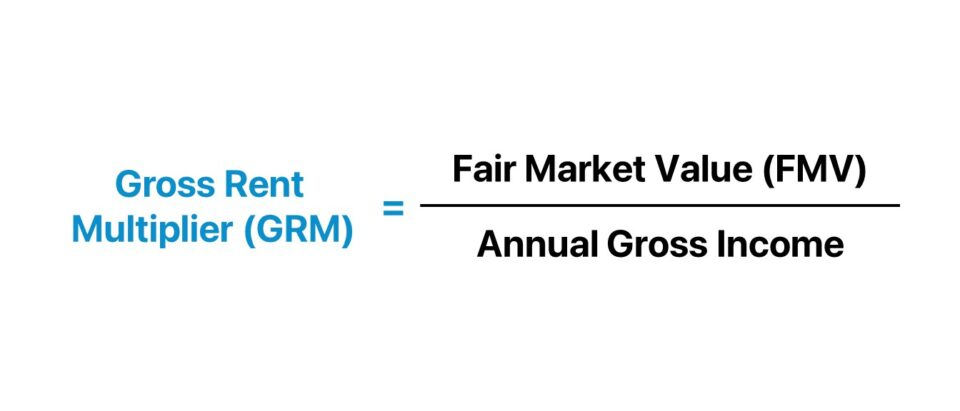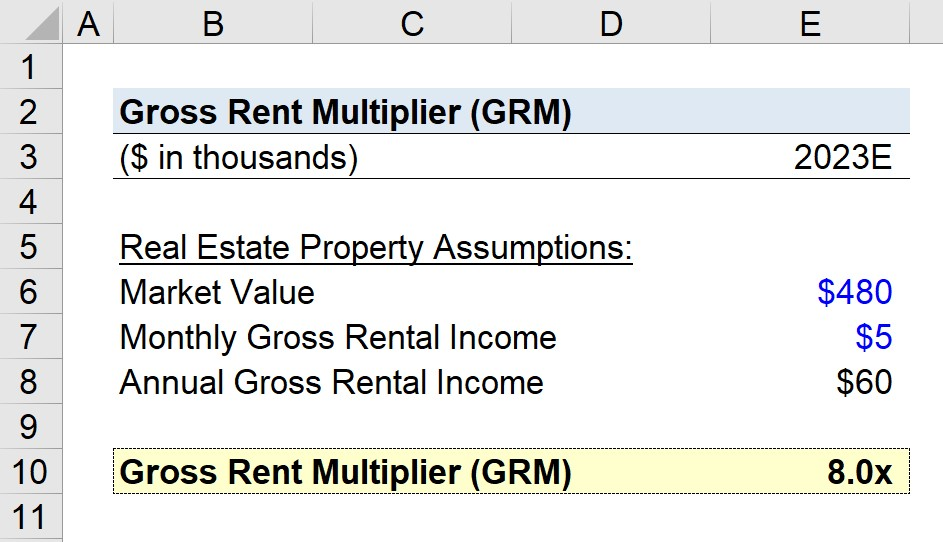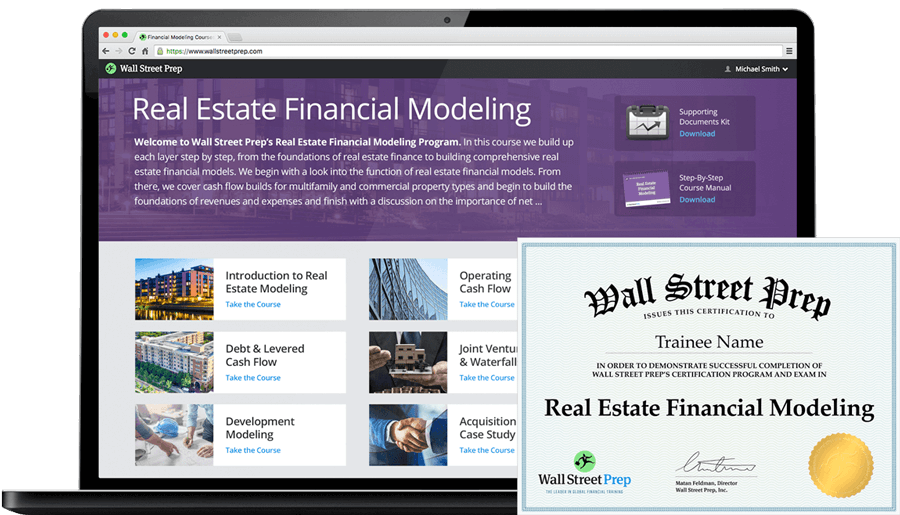Welcome to Wall Street Prep! Use code at checkout for 15% off.# Gross Rent Multiplier (GRM)

Guide to Understanding the Gross Rent Multiplier (GRM)## How to Calculate Gross Rent Multiplier?

The gross rent multiplier (GRM), or “gross rental multiplier”, reflects the number of years it would take for a particular property’s gross rental income to pay for itself.

Most often, the gross rent multiplier (GRM) metric is used by real estate investors and other market participants to ensure that a potential property investment can in fact become profitable.

In practice, the gross rental multiplier is more of a screening tool – i.e. a “quick and dirty” method – to determine the potential profitability of a real estate investment.

Therefore, the gross rental multiplier (GRM) is not only useful for screening purposes, but also for assessing comparable properties.

The multiplier illustrates the “big picture” in terms of the property’s profit potential, enabling real estate investors to determine whether a property investment generates sufficient rental income to justify an investment in it.

To calculate the gross rental multiplier (GRM) metric, only two inputs are necessary:

1. Property Value → The fair market value (FMV) of the property as of the present date, i.e. the asking price at which the property can be purchased.
2. Gross Annual Income → The estimated amount of rental income expected to be produced each year.

From those two figures, dividing a property’s fair value by its gross annual income yields the gross rental multiplier (GRM).## Gross Rent Multiplier Formula (GRM)

The formula for calculating the gross rent multiplier (GRM) is as follows.

Gross Rent Multiplier (GRM) = Fair Market Value (FMV) ÷ Annual Gross Income

For example, suppose a property’s current fair value is \$300k, while its annual gross income is projected to be \$60k.

Given those assumptions, we can calculate the gross rent multiplier as 5.0x.

• Gross Rent Multiplier (GRM) = \$300k ÷ \$60k = 5.0x

The 5.0x multiple suggests that for the property to break even, it would take approximately five years.

## Gross Rent Multiplier Calculator (GRM)

We’ll now move to a modeling exercise, which you can access by filling out the form below.#### Excel TemplateSubmitting...

## 1. Real Estate Property Rental Income Calculation Example

Suppose a real estate investor is considering purchasing a multifamily property priced at \$480k near the end of 2022.

The monthly rent charged to the future tenants is expected to be \$5,000 in total.

In order to annualize our monthly rental income, we must multiply the monthly gross income by 12.

• Monthly Gross Rental Income = \$5k
• Annual Gross Rental Income = \$5k × 12 = \$60k

The property investment will generate roughly \$60k per year.

## 2. Gross Rent Multiplier Calculation Example (GRM)

In the next section, we’ll divide the property’s fair value by the gross annual income of the property to calculate the gross rent multiplier (GRM).

• Gross Rent Multiplier (GRM) = \$480k ÷ \$60k = 8.0x

In conclusion, the 8.0x gross rent multiplier (GRM) implies that the multifamily real estate property investment should take approximately eight years for the investor to recoup the initial investment and become profitable.## What is a Good Gross Rent Multiplier?

As a general rule of thumb, the lower the gross rent multiplier (GRM), the more profitable the property is likely to be (and vice versa).

Why? A lower gross rental multiplier (GRM) implies a shorter payback period and greater upside potential in terms of deriving more profits over time.

Therefore, the lower the gross rent multiplier (GRM), the higher the expected rate of return (i.e. yield) received by the real estate investor.

## GRM vs. Cap Rate: What is the Difference?

The capitalization rate, or “cap rate” for short, compares a rental property’s net operating income (NOI) to its fair value. Like the GRM, the cap rate is also used to evaluate returns and profitability in real estate.

Cap Rate (%) = Net Operating Income (NOI) ÷ Property Value

Contrary to the gross rent multiplier (GRM), the higher the cap rate, the higher the expected return on investment (ROI) – all else being equal.

The net operating income (NOI), a critical input when calculating the cap rate, subtracts various types of operating expenses such as unit repairs, vacancies, property taxes, and insurance.

Therefore, the capitalization rate is considered to be a more comprehensive, informative metric in real estate investing, but also more time-consuming to calculate. The GRM, however, is primarily used as a screening tool.20+ Hours of Online Video Training

### Master Real Estate Financial Modeling

This program breaks down everything you need to build and interpret real estate finance models. Used at the world's leading real estate private equity firms and academic institutions.

Inline Feedbacks